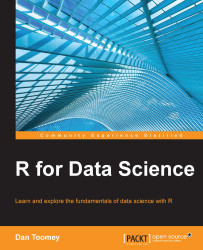•#### R for Data Science#### Overview of this book

R for Data ScienceCreditswww.PacktPub.comPrefaceFree Chapter
Data Mining PatternsData Mining SequencesText MiningData Analysis – Regression AnalysisData Analysis – CorrelationData Analysis – ClusteringData Visualization – R GraphicsData Visualization – PlottingData Visualization – 3DMachine Learning in ActionPredicting Events with Machine LearningSupervised and Unsupervised LearningIndex## Summary

In this chapter, we discussed how to perform regression analysis using R. We performed simple regression and analyzed fit, residuals, and other factors. We used multiple regression, including selecting and using a set of predictor values. We tried to determine a set of values from predictors using multivariate regression. Lastly, we used robust regression to overcome possible problems in the predictors and to build a reliable model. In the next chapter, we will be covering correlation.